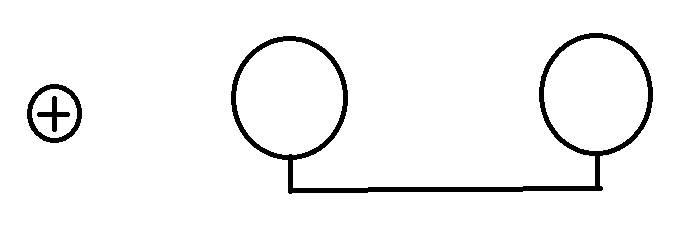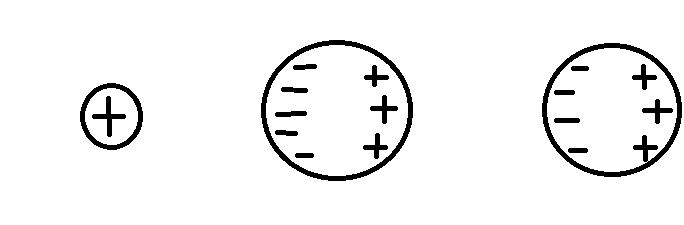# How does charge distribute on connected conductors

• T. Centa
I'm still unable to express these quantities mathematically. Does it use the method of images? I still haven't learned how to use...f

#### T. Centa

This is a question that is vaguely related to coursework but is more for curiosity.
Lets say there are two spheres joined by a thin wire in the presence of an electric field:Assume the conductors initially have no charge. Now I am pretty sure without the wire, the charge distribution would look something like this:But for the life of me I cannot figure out how it is spread when they are joined by a wire because I am not sure how having the same potential would affect their charge distributions. Any help on this would be appreciated.

Not sure whether you are asking if there would be any redistribution, or you realize that there will be but do not know how to determine it.

I too had this doubt while studying electromagnetism. Still can't figure it outAnyone?

I too had this doubt while studying electromagnetism. Still can't figure it outAnyone?
A key question is whether you can ignore the field at each sphere that arises from the charges on the other sphere. If you cannot then even with no external field (the + charge on the left in the diagram at the start of this thread) this is quite a tricky problem. See https://arxiv.org/pdf/0906.1617.pdf.
If you can ignore the interaction of the fields from the spheres, you can treat each sphere independently as a charged conducting sphere immersed in a uniform field (parallel plates, say) and find its potential as a function of charge and radius. Then set the two potentials as equal.

A key question is whether you can ignore the field at each sphere that arises from the charges on the other sphere.
This was actually my original doubt while studying potentials; that if two charged metal spheres are in the vicinity of each other what would the potential of each be..
Thanks for the link. The paper looks a bit long. I'll read it in some time, have an exam coming up.

This was actually my original doubt while studying potentials; that if two charged metal spheres are in the vicinity of each other what would the potential of each be..
Thanks for the link. The paper looks a bit long. I'll read it in some time, have an exam coming up.
To whet your appetite, as I recall, it is possible for two spheres with the same sign of net charge to attract(!)

To whet your appetite, as I recall, it is possible for two spheres with the same sign of net charge to attract(!)
Really?! That's counterintuitive. I'll look into it as soon as I get time.

Really?! That's counterintuitive. I'll look into it as soon as I get time.
Consider the case of one strongly charged sphere and one neutral sphere. You agree that the induced charge separation on the neutral sphere results in an attraction, right? Now put a tiny net charge on the "neutral" sphere -- so tiny that the net force is still an attraction.

Voila. Two spheres with the same [signed] net charge and a net attraction.

Last edited:
•Delta2
This is a question that is vaguely related to coursework but is more for curiosity.

But for the life of me I cannot figure out how it is spread when they are joined by a wire because I am not sure how having the same potential would affect their charge distributions. Any help on this would be appreciated.

The two spheres are not in the same potential BEFORE we attach the wire. The left sphere that is closest to the source charge + has higher potential ##C_1## than the right sphere ##C_2##.

When we connect the wire the surface charges redistribute so that the potential becomes everywhere (on the conducting structure consisting of the spheres and the wire) the same C, ##C_2<C<C_1##. We can easily understand why this happens if we view the structure as a unified conducting object. However I admit if we see the structure in sequential steps our intuition is bugging.

Consider the case of one strongly charged sphere and one neutral sphere. You agree that the induced charge separation on the neutral sphere results in an attraction, right? Now put a tiny net charge on the "neutral" sphere -- so tiny that the net force is still an attraction.

Voila. Two spheres with the same [signed] net charge and a net attraction.
Yes, this was exactly my thought process. It makes sense here. But can the two spheres attract even if they have charges of equal magnitude? Attraction in this case , if it happens, seems against intuition.
When we connect the wire the surface charges redistribute s that the potential becomes everywhere (on the conducting structure consisting of the spheres and the wire) the same ##C, C_2<C<C_1##
I'm still unable to express these quantities mathematically. Does it use the method of images? I still haven't learned how to use that.

The two spheres are not in the same potential BEFORE we attach the wire. The left sphere that is closest to the source charge + has higher potential ##C_1## than the right sphere ##C_2##.

When we connect the wire the surface charges redistribute so that the potential becomes everywhere (on the conducting structure consisting of the spheres and the wire) the same C, ##C_2<C<C_1##. We can easily understand why this happens if we view the structure as a unified conducting object. However I admit if we see the structure in sequential steps our intuition is bugging.
I may be wrong, but I think T.Centa is asking how to compute the new charge distribution.

I may be wrong, but I think T.Centa is asking how to compute the new charge distribution.

Yes I guess so now that I read OP again.